# The Poisson top

The Poisson top, a close relative to the Lagrange top, is an axisymmetric rigid body that is free to move on a horizontal surface with one point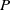in contact with the ground, as illustrated in Figure 1 below. In the early 1800s, Poisson  examined the dynamics of this top in the (overly optimistic) hopes of being able to use it to establish the vertical (i.e., the plumb line) in a ship at sea.

## Equations of motion

Following our discussion of the Lagrange top, the Poisson top depicted in Figure 1 has mass, a longitudinal axis of symmetry, and a center of mass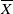that falls along this axis such that the top’s principal moments of inertiaand. The position of the moving contact pointrelative to the mass center is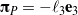, where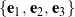is the top’s corotational basis. We locate the top’s center of mass using a set of Cartesian coordinates,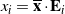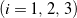, where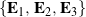denotes the space-fixed basis, while the rotation of the top may be parameterized by a set of Euler angles: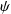,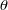, and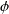.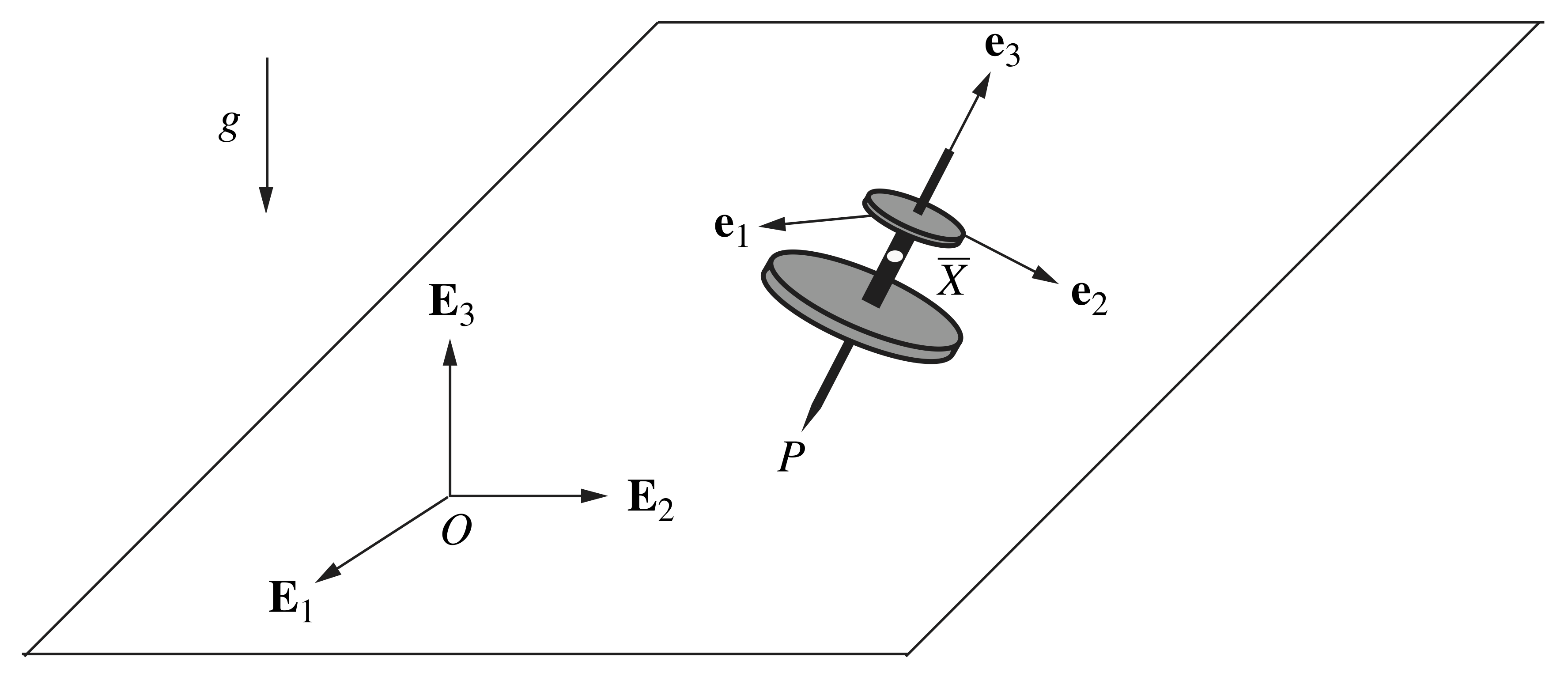Figure 1. Schematic of the Poisson top illustrating the alignment of the corotational basis .

In contrast to the Lagrange top, because the Poisson top’s contact pointis able to slide on a horizontal surface, the top is subject to a single integrable (i.e., holonomic) constraint given by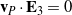; that is, pointmaintains contact with the horizontal surface, and thus it does not have a vertical component of velocity.

The equations of motion for the Poisson top may be obtained by a Lagrangian approach, for which the top’s potential energy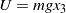, and its total kinetic energy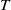has the form

(1)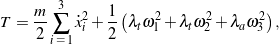where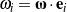are the corotational components of the top’s angular velocity. In terms of a 3-2-3 set of Euler angles,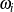are related to,, andand their rates of change according to the following expressions:

(2)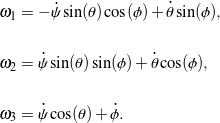We assume the horizontal surface over which the Poisson top slides is smooth. Upon applying Lagrange’s equations, it can be shown that the top’s equations of motion for the unconstrained coordinatesmay be expressed in the second-order matrix-vector form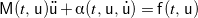, where

(3)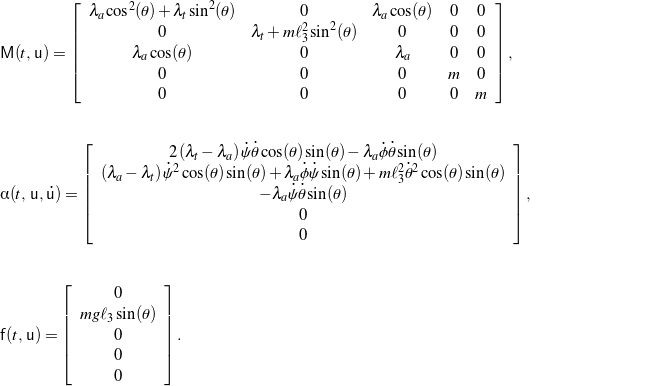In order to numerically integrate the top’s equations of motion in MATLAB, we introduce the state vector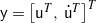so that the state-space (i.e., first-order) formulation of the second-order equations of motion is given by

(4)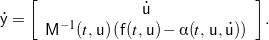## Simulation and animation

A sample animation of the Poisson top’s motion obtained by numerical simulation is provided in Figure 2. The animation window also displays the evolution of the corotational basis vectors, the trajectory of the top’s contact point, and the total mechanical energy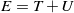over time.

Note that, as with the Lagrange top, the Poisson top can execute a steady rotational motion with a constant precession rate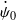, spin rate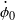, and nutation angle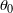if they satisfy the condition

(5)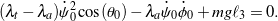Indeed, Figure 3 demonstrates one such steady motion. Notice that the path of the top’s contact pointtraces out a circle in this case, and this is true of all steady motions.

Lastly, we wish to point out that because the top is allowed to slide without friction, its motion conserves not only the total mechanical energy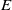, but also the lateral components of the top’s linear momentum,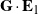and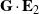. In addition, the top’s motion conserves two components of the angular momentum with respect to the mass center: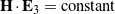and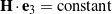.